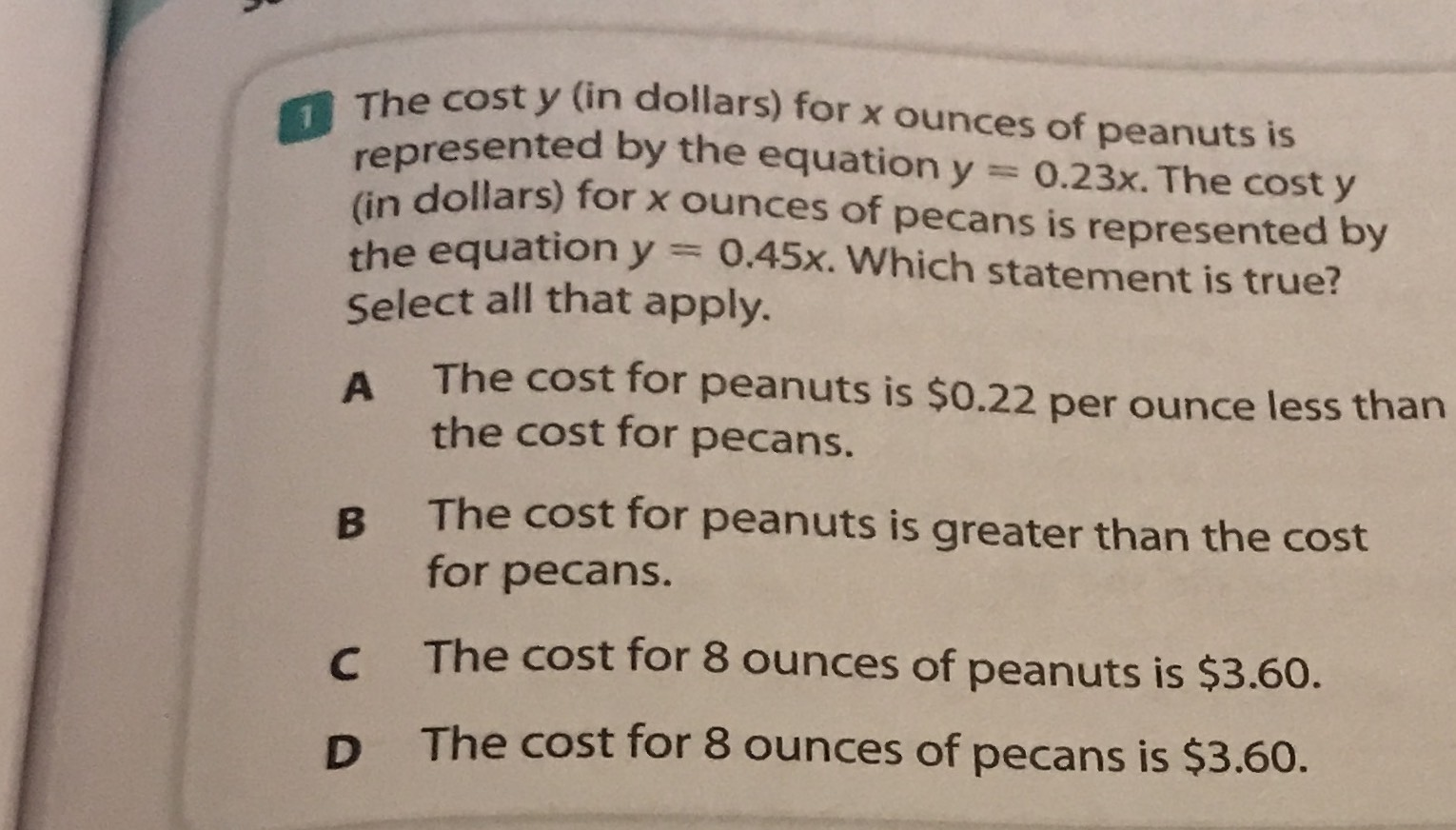### ¿Todavía tienes preguntas de matemáticas?

Pregunte a nuestros tutores expertos
Algebra
Pregunta$$1$$ The cost $$y$$ (in dollars) for $$x$$ ounces of peanuts is represented by the equation $$y = 0.23 x$$ . The cost $$y$$ (in dollars) for $$x$$ ounces of pecans is represented by the equation $$y = 0.45 x$$ . Which statement is true? Select all that apply.

A The cost for peanuts is $$\ 0.22$$ per ounce less than the cost for pecans.

B The cost for peanuts is greater than the cost Cor pecans.

C The cost for $$8$$ ounces of peanuts is $$\ 3.60$$ .

D The cost for $$8$$ ounces of pecans is $$\ 3.60 .$$